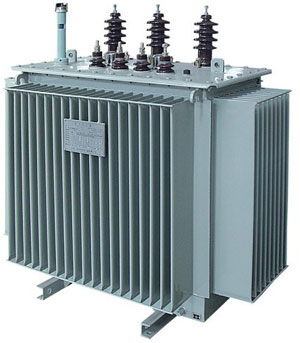Categories

# Current Transformer Secondary Connection

In circuit theory, current through two series resistors is the same even if the resistors are of different resistances. It's the voltage across the resistors that is different. Look at reactors/capacitors, and it's the same thing. Now, current through parallel resistors is different through each resistor, as the current divides among the parallel resistors. So, put the meters in series on the secondary of a current transformer.

Now, whether one CAN put two of them in series, versus only one on the current transformer secondary depends largely on the current transformer. If the burden to the current transformer is low enough (the impedance connected to the current transformer secondary), the current transformer can accurately supply current to the meters that is a good representation of the primary current.Note that this has nothing to do with current lost in a series connection (which doesn't happen, anyway) to the resistance of the meter, but it has to do with the capability of the current transformer to supply an accurate waveform of current to the meters; this is due to the current transformer having to "use" more primary current for excitation current that generates a large enough voltage on the secondary terminals. What the current transformer excitation current is doing, essentially, is generating flux in the core of the transformer. There is an upper limit to how much flux there can be in the core before it saturates and the secondary current no longer is an accurate enough reproduction of the primary current. Too much excitation current, which is basically shunting away the available secondary current from the meters, will leave too little secondary current/distorted secondary current for the meters to measure properly. The impedance of the meters and their leads connected across the secondary terminals of a current transformer is so large that the current transformer has to produce too much voltage to drive current through them accurately...it saturates in this case. The extreme of this condition is an open secondary of a current transformer that has, theoretically, infinite impedance. In that case the voltage produced on the current transformer secondary will reach large peaks until the current transformer saturates on each half cycle.

So, the meters should be connected in series on the secondary of a current transformer, and the "minus the current lost to the resistance of the meter, plus each connection point" may be ignored, because that's not what's going on in the circuit. The important part is calculating to see if the current transformer can handle the burden the meters, leads, connection points, current transformer secondary winding impedance presents to the current transformer. If a current transformer with a large enough core is used, it can handle a relatively great amount of burden. So, I'll say this for emphasis, it's an instrument transformer problem, not a problem with the meters...directly. The burden the meters present to the current transformer is a problem. Many times, people not familiar with meters will automatically assume meters cannot be connected in series on a current transformer (some facilities/utilities require them to be on their own current transformer, possibly being the source of this misconception), but indeed they can. Of course, a watt-hour meter needs a voltage input, and they are connected in parallel on the voltage transformer secondary. The voltage transformer secondary is limited to the amount of VA it can supply its burden....which can be great depending on the voltage transformer selected. So, inaccurate metering is mitigated in several ways, among them the selection of the instrument transformer ratings or reducing the connected secondary burden to the instrument transformer in some manner...through maybe using a meter with less burden or using larger conductor for the leads or shortening the lead length somehow.

Transformer